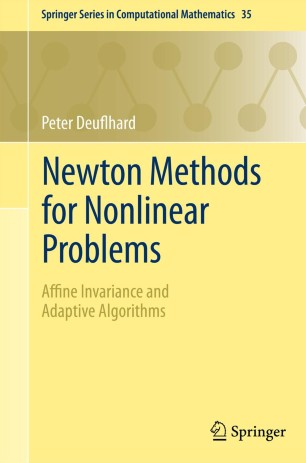# Newton Methods for Nonlinear Problems

## Affine Invariance and Adaptive Algorithms

• Peter DeuflhardTextbook

Part of the Springer Series in Computational Mathematics book series (SSCM, volume 35)

1. Front Matter
Pages i-xii
2. Peter Deuflhard
Pages 7-41
3. ### Algebraic Equations

1. Front Matter
Pages 43-43
2. Peter Deuflhard
Pages 45-107
3. Peter Deuflhard
Pages 109-172
4. Peter Deuflhard
Pages 173-231
5. Peter Deuflhard
Pages 233-282
4. ### Differential Equations

1. Front Matter
Pages 283-283
2. Peter Deuflhard
Pages 285-314
3. Peter Deuflhard
Pages 315-368
4. Peter Deuflhard
Pages 369-404
5. Back Matter
Pages 405-424

### Introduction

This book deals with the efficient numerical solution of challenging nonlinear problems in science and engineering, both in finite dimension (algebraic systems) and in infinite dimension (ordinary and partial differential equations). Its focus is on local and global Newton methods for direct problems or Gauss-Newton methods for inverse problems. The term 'affine invariance' means that the presented algorithms and their convergence analysis are invariant under one out of four subclasses of affine transformations of the problem to be solved. Compared to traditional textbooks, the distinguishing affine invariance approach leads to shorter theorems and proofs and permits the construction of fully adaptive algorithms. Lots of numerical illustrations, comparison tables, and exercises make the text useful in computational mathematics classes. At the same time, the book opens many directions for possible future research.

### Keywords

Gauss-newton methods Newton methods affine invariance continuation methods differential equations

#### Authors and affiliations

• Peter Deuflhard
• 1
1. 1.Zuse-Institut Berlin (ZIB)BerlinGermany

Industry Sectors